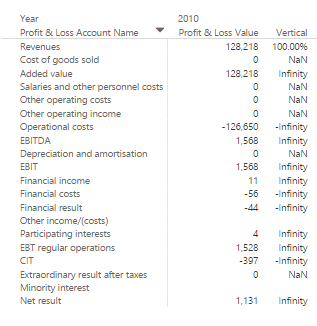cancel
Showing results for
Did you mean:Helper I

## PnL: Vertical Analysis % issue

All,

I need to implement vertical analysis to my PnL statement.

My DAX query to calcualte the percentage is as follows:

Vertical = 'Profit & Loss Measures 1 Company'[Profit & Loss Value]/(CALCULATE(SUM('Profit & Loss Statement'[Profit & Loss Balance]),FILTER('Profit & Loss Statement','Profit & Loss Statement'[Profit & Loss Account Number]=1)))

End result of the above expression is:So a lot of NaN and Infinity entries.

However, if I replace the second part of the expression (calculate.....) with a static number, say 2010 Revenue value 128,218, the the Vertical expression get evaluated correctly and correct percentages are displayed.Any idea how to resolve this with the CALCUALTE function? I need this to be a dynamic value.

Thanks.

7 REPLIES 7Microsoft

@mapko

If the result of following DAX measure is 0 or BLANK(), you will get NaN and Infinity entries for Vertical. Just to confirm, is it as expected that you get 0 or BLANK() with following formula in some rows?

```CALCULATE (
SUM ( 'Profit & Loss Statement'[Profit & Loss Balance] ),
FILTER (
'Profit & Loss Statement',
'Profit & Loss Statement'[Profit & Loss Account Number] = 1
)
)
)```

0 / BLANK () = NaN

0 / 0 = NaN

4 / BLANK () = Infinity

10 / 0 Infinity

Best Regards,

HerbertHelper I

@v-haibl-msft, thanks for your reply. The expectation is that the DAX measure always returns sum of the Revenue account and then divide each account within the PnL statement with this measure.

Thank you!Microsoft

@mapko

Is the sum of the Revenue account a dynamic value in different rows? For example, for Revenues row, its value is 128218, and for Cost of goods sold row, its value is 137317. The result of Vertical is 128218/128218 and 0/137317 for the first two rows? In that case, we need to know the calculation method of sum of the Revenue account. You’d better give some sample data of your table and the expected output.

Best Regards,

HerbertHelper I

@v-haibl-msft

I actually resolved this. Happy to share my expression if its interesting for you.

MarkoMicrosoft

@mapko

I would be great if you can share your expression. You can share it and then mark it as solution. It is helpful to others if they have the same problem.

Best Regards,

HerbertSuper User

If you put the second part of your formula in your measure/column, what do you get? Seems like it is caculating to zero.

`(CALCULATE(SUM('Profit & Loss Statement'[Profit & Loss Balance]),FILTER('Profit & Loss Statement','Profit & Loss Statement'[Profit & Loss Account Number]=1)))`

What happens when you take out the filter?

Any chance you can share some sample data?

Become an expert!: Enterprise DNA
External Tools: MSHGQM
Latest book!:
Mastering Power BI 2nd EditionDAX is easy, CALCULATE makes DAX hard...Helper I
For the revenue line it calculates the correct value. Other accounts are blank.